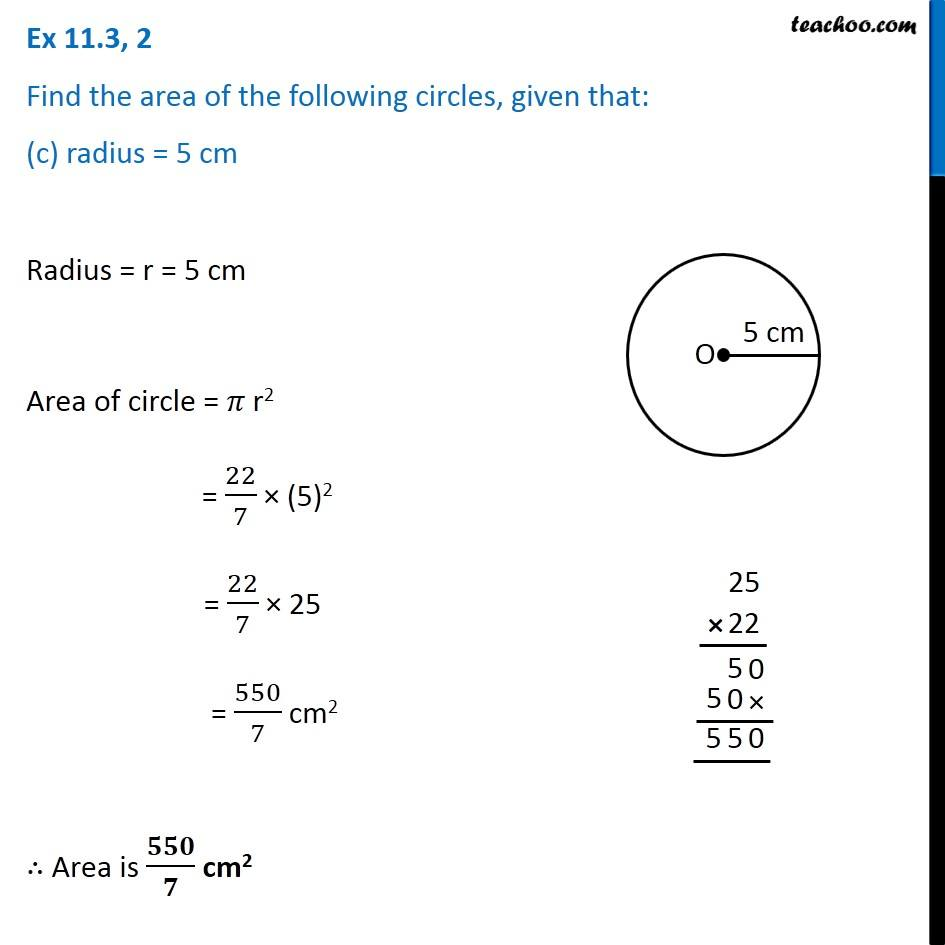Ex 11.3

Chapter 11 Class 7 Perimeter and Area
Serial order wiseIntroducing your new favourite teacher - Teachoo Black, at only ₹83 per month

### Transcript

Ex 11.3, 2 Find the area of the following circles, given that: (c) radius = 5 cm Radius = r = 5 cm Area of circle = 𝜋 r2 = 22/7 × (5)2 = 22/7 × 25 = 550/7 cm2 ∴ Area is 𝟓𝟓𝟎/𝟕 cm2